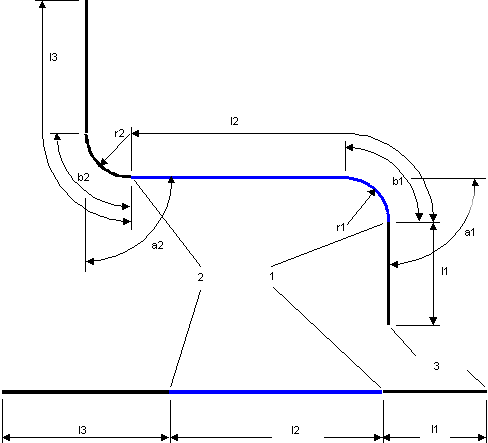Bending point is the point where the bending starts. The distance between two bending points or the distance between a bending point and a pipe end represents the bending point.
Theoretical bending point—The bending point that is derived directly from a pipe 3D model as shown in the following figure.1. First bending point
2. Second bending point
a(n) Bending angle
b(n) Bending length
l(n) Bending point location